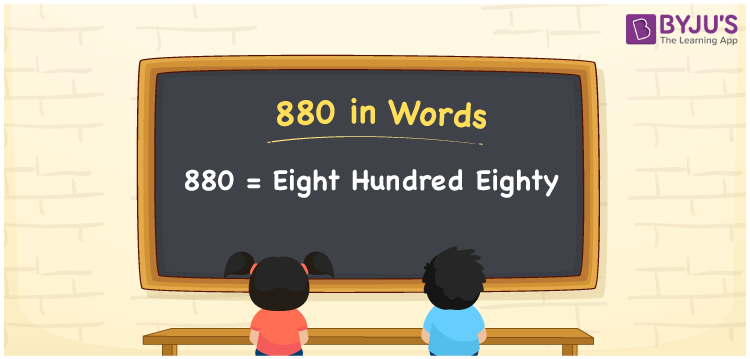# 880 in words

880 in words is written as Eight Hundred and Eighty. 880 represents the count or value. The article on Counting Numbers can give you an idea about count or counting. The number 880 is used in expressions that relate to money, days, distance, length, weight and so on. Let us consider an example for 880. “A 2 year old Rino weighs around Eight Hundred and Eighty Kilos.” Another example, “A new born baby weighing only Eight Hundred and Eighty grams required four months of intensive care for recovery.”

 880 in words Eight Hundred and Eighty Eight Hundred and Eighty in Numbers 880

## 880 in English Words## How to Write 880 in Words?

We can convert 880 to words using a place value chart. The number 880 has 3 digits, so let’s make a chart that shows the place value up to 3 digits.

 Hundreds Tens Ones 8 8 0

Thus, we can write the expanded form as:

8 × Hundred + 8 × Ten + 0 × One

= 8 × 100 + 8 × 10 + 0 × 1

= 880

= Eight Hundred and Eighty.

880 is the natural number that is succeeded by 879 and preceded by 881.

880 in words – Eight Hundred and Eighty.

Is 880 an odd number? – No.

Is 880 an even number? – Yes.

Is 880 a perfect square number? – No.

Is 880 a perfect cube number? – No.

Is 880 a prime number? – No.

Is 880 a composite number? – Yes.

## Solved Example

1. Write the number 880 in expanded form

Solution: 8 × 100 + 8 × 10 + 0 × 1

We can write 880 = 800 + 80 + 0

= 8 × 100 + 8 × 10 + 0 × 1

## Frequently Asked Questions on 880 in words

Q1

### How to write 880 in words?

880 in words is written as Eight hundred and Eighty.
Q2

### State True or False. 880 is divisible by 2?

True. 880 is divisible by 2.
Q3

### Is 880 a perfect square number?

No. 880 is not a perfect square number.